Peer review of ICSE Class 9 Maths Solutions S Chand Chapter 11 Rectilinear Figures Chapter Test can encourage collaborative learning.

## S Chand Class 9 ICSE Maths Solutions Chapter 11 Rectilinear Figures Chapter Test

Question 1.
Which of the following terms best describes the figure below?
(a) Rhombus
(b) Trapzoid
(d) Square
(e) Parallelogram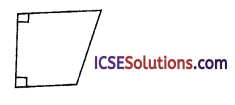Solution:
(b) Trapzoid
The given figure is a trapezium.
∵ A pair of two opposite sides are parallel.

Question 2.
Give the quadrilateral below, what value of x would allow you to conclude that the figure is a parallelogram?(a) – 2
(b) 0
(c) 1
(d) 2
(e) 3
Solution:
(e) 3
∵ The given figure is of a parallelogram.
∴ Their opposite sides are equal and parallel
∴ x – 1 = 2x – 4 ⇒ – 1 + 4 = 2x – x ⇒ x = 3
2x + 1 = 3x – 2 ⇒ 3x – 2x = 1 + 2 ⇒ x = 3
∴ x = 3Question 3.
In the figure, if ABCD is a rectangle, what type of triangle must ∆ABD be?(a) Equilateral
(b) Right
(c) Equiangular
(d) Isosceles
(e) Scalene
Solution:
(b) Right
In figure, ABCD is a rectangle whose diagonals bisect each other at O.
∆ABD is a right triangle as ∠A = 90°.

Question 4.
Find the measures of the lattered angles in each figure.
(i) ABCD is a parallelogram.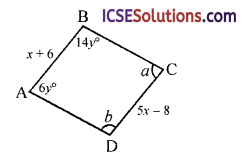(ii) rectangle PQRS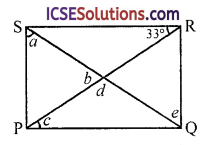(iii) rhombus MNPQ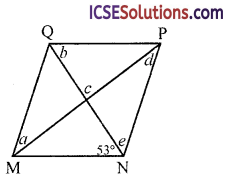Solution:
(i) In figure, ABCD is a parallelogram
∴ Their opposite sides are equal and parallel and opposite angles are equal
∴ x + 6 = 5x – 8 ⇒ 5x – x = 6 + 8
⇒ 4x = 14 ⇒ x = $$\frac { 14 }{ 4 }$$ = 3.5 4
⇒ 14y + 6y = 180 (co-interior angles)
20y = 180° ⇒ y = $$\frac { 180° }{ 20° }$$ = 9
6y = a ⇒ 6 x 9 = a ⇒ a = 54°
b = 14y = 14 x 9 = 126°
∴ a = 54°, b = 126°

(ii) PQRS is a rectangle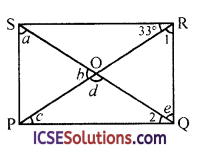∴ Opposite sides are equal and parallel. Diagonals bisect each other
c = 33° (Alternate angles)
Similarly, a = e (Alternate angles)
e = ∠1 (∵ OQ = OR)
and ∠1 + 33° = 90°
⇒ ∠1 = 90° – 33° = 57°
∴ e = ∠1 = 57° and a = e = 57°
∵ OP = OQ
∴ c = ∠2 = 33°
d = 180° – c – ∠2 = 180° – 33° – 33°
= 180 – 66° = 114°
and b + d = 180°
⇒ b = 180° – d = 180°- 114° = 66°
Hence,
a = 57°, b = 66°, c = 33°, d = 114°, e = 57°(iii) MNPQ is a rhombus in which all sides are equal and diagonals bisect each other at 90° and diagonals bisect opposite angles
b = 53° (alternate angles)
a = d (alternate angles)
e = 53°
but e + d = 90° (in ∆PON)
53° + d = 90° ⇒ d = 90° – 53° = 37° a = d = 31°
∠c = 90°
(∵ Diagonals bisect each other at 90°)
a = 37°, b = 53°, ∠c = 90°, d = 37°, e = 53°

Question 5.
In kite ABCD, ∠OBC = 58°, and ∠DAB = 50°, and the measures of(i) ∠BCD
(ii) ∠DAO
(iii) ∠ODA
Solution:
In the figure, ABCD is a kite in which BC = CD, AB = AD
and diagonals AC and BD intersect at right angles at O and AC bisects the oppoiste angles.
∠OBC = 58°, ∠DAB = 50°(i) In ABCD, BC = CD
∴ ∠OBC = ∠ODC = 58°
∴ ∠BCD = 180° – ∠OBC – ∠ODC
= 180° – 58° – 58°
= 180°- 116° = 64°

(ii) ∠DAO = $$\frac { 1 }{ 2 }$$ ∠BAD = $$\frac { 1 }{ 2 }$$ x 50° = 25°

(iii) ∠ODA = 90° – ∠DAO = 90° – 25° = 65°

(iv) ∠ADC = ∠ODA + ∠ODC = 65° + 58° = 123°

Question 6.
Given: PQ and QR are mid segment of ∆ABC and AB = BC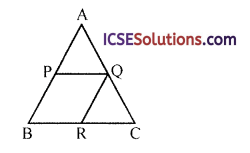Prove: BPQR is a rhombus.
Solution:
In ABC, PQ and QR are mid segments AB = BC
∴ PQ || BC and PQ = $$\frac { 1 }{ 2 }$$ BC = BR … (i)
(R is mid point of BC)
But AB = BC and P, R their mid points
∴ PB = BR … (ii)
and QR || AB and QR = $$\frac { 1 }{ 2 }$$ AB = PB
From (i), (ii) and (iii),
PB = BR = QR = PQ
∴ BPQR is a rhombus or a square
But ∠B ≠ 90°
∴ BPQR is a rhombus.